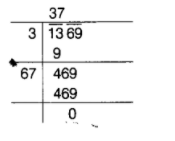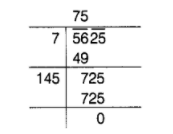# Find the square root of the following

Question:

Find the square root of the following by long division method.

(a) 1369

(b) 5625

Solution:

(a) We have, 1369$\therefore \quad \sqrt{1369}=37$

(b) We have, 5625$\therefore \quad \sqrt{5625}=75$